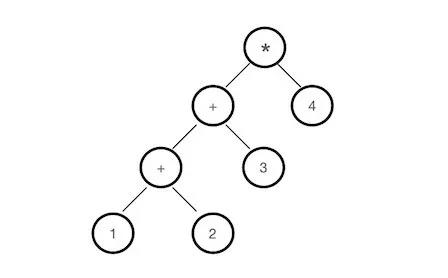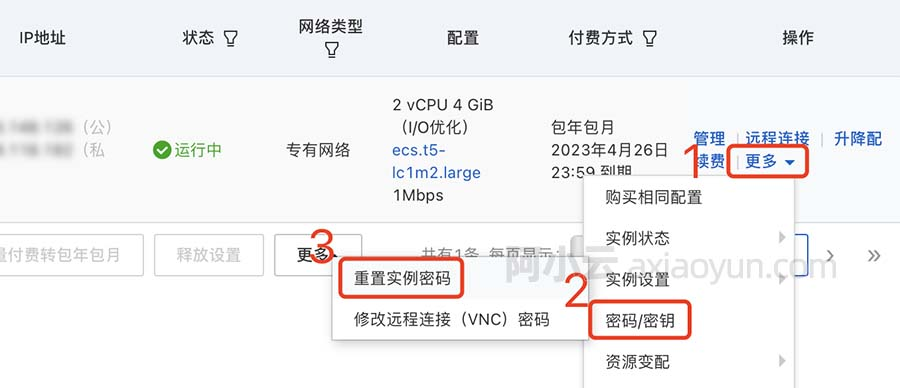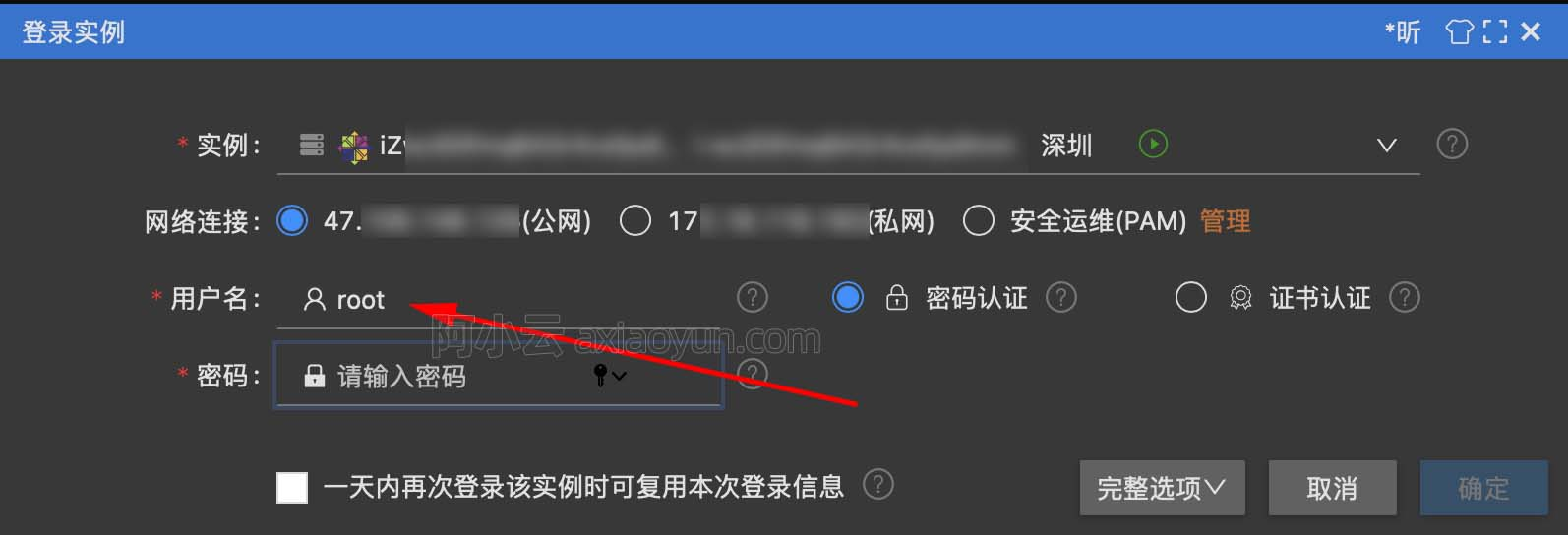Swift 与 OC 枚举的异同

+关注继续查看Swift 与 OC 枚举存在的不同问题与解决方案。

OC 中枚举的问题

typedef NS_ENUM(NSUInteger, Direction) {
EAST,
SOUTH,
WEST,
NORTH
};

if (direction == EAST) {
// do something
}

if (direction == 0) {
// do something
}

Swift 中的枚举

Swift中的枚举变成了一个独立的类型，这意味着，当我们在Swift中同样的定义：

enum Direction {
EAST,
SOUTH,
WEST,
NORTH
};

Swift为枚举提供了远超整型的能力，除了基本的if和switch模式匹配等基本用法之外，还可以：

1. 指定成员的类型，如Int、String以及任意的类型
2. 定义方法和计算属性
3. 获取枚举所有成员的数组，Direction.allCases.
4. 关联值，可以使用元组来存储数据，配合模式匹配可以大幅减少代码，并且支持泛型关联值。
5. 作为一个类型，可以实现协议，定义扩展
6. 实现递归枚举，可以很方便的表示类似列表或者树的结构，配合递归函数降低理解成本。

Swift中的枚举和OC枚举的相同之处就是名称一样，内涵和外延已经完全不同，其核心价值在于提供了在多种类型（而非仅仅整型）间做选择的机制，在将这种机制的实现细节与对外抽象分离的同时，赋予其上述所有的能力，这提供了更高的抽象层次和更强的表现能力。

func makeModel1(s : String?) -> Model?
{
let d1 = s?.data(using: String.Encoding.utf8)

// 判断是否为空
if let a = d1 {
// 可能throw的代码try一下
guard let dict  = try? JSONSerialization.jsonObject(with: a) as? Dictionary<String, String> else {
fatalError("Json data error")
}
let model = Model.init(dict: dict)
return model
}

return nil
}

func makeModel2(s : String?) -> Model?
{
s?.data(using: String.Encoding.utf8).map{
try? JSONSerialization.jsonObject(with: $0) }.map { Model.init(dict:$0 as! Dictionary<String, String>)
}
}

func makeModel2(s : String?) -> Model?
{
s?.data(using: String.Encoding.utf8).map{try? JSONSerialization.jsonObject(with: $0)}.map { Model.init(dict:$0 as! Dictionary<String, String>)}
}1. 表达式可以体现为树的形式，而树是很适合用递归来描述的；
2. 表达式的节点分为两种，一种是数字，一种是运算，并且每个运算必定关联两个节点
3. 表达式的求值过程式是递归地将运算应用到两个节点的过程

enum Exp {
case value(Double)            //值节点
indirect case plu(Exp, Exp)    //加法
indirect case sub(Exp, Exp)    //减法
indirect case mul(Exp, Exp)    //乘法
indirect case div(Exp, Exp)    //除法

// 求值，模式匹配
func eval() -> Double {
switch self {
case .value(let value):
return value;
case .plu(let exp1, let exp2):
return exp1.eval() + exp2.eval()
case .sub(let exp1, let exp2):
return exp1.eval() - exp2.eval()
case .mul(let exp1, let exp2):
return exp1.eval() * exp2.eval()
case .div(let exp1, let exp2):
return exp1.eval() / exp2.eval()
}
}
}

1. 将4个数字表示为可能的表达式数组，这一步又分成两小步：
获取4个数字的全排列针对每一种排列方式，在每两个相邻的数字中间插入不同的运算符号
2. 对数组中的表达式求值，取其中结果为24的表达式

// 查找4个数字的24点答案
func search24(_ nums:(Double, Double, Double, Double)) -> [Exp] {
return makeExpFromNums([nums.0,nums.1,nums.2,nums.3]).filter{ $0.eval() == 24} } // 将一个数组递归构造成Exp的数组 func makeExpFromNums(_ nums:[Double]) -> [Exp] { if (nums.count == 0) { //返回空数组 return [] } else if (nums.count == 1) { // 返回值 return [.value(nums.first!)] } else { // 1、排列，2、分割数组，3、对分割后的两部分递归构造Exp，4、对递归的两个Exp数组组装成最终的Exp数组 return permutation(nums).flatMap{partition($0).flatMap{makeExpFromExpArray((makeExpFromNums($0.0), makeExpFromNums($0.1)))}}
}
}

// 构造4个数字的全排列，这是个递归函数，将第一个元素插入到后续元素的全排列中即可
func permutation(_ nums:[Double]) -> [[Double]] {
if (nums.count == 0) {
return [[]]
}
return permutation(Array<Double>(nums.suffix(from: 1))).flatMap{insert(num: nums.first!, nums: $0)} } // 给点一个数组nums，有nums.count+1的插入点 func insert(num:Double, nums:[Double]) -> [[Double]] { (0...nums.count).map{nums.prefix(upTo:$0) + [num] + nums.suffix(from:$0)} } // 将一个数组分割成两部分 func partition(_ nums:[Double]) -> [([Double], [Double])] { (0...nums.count).map{(Array(nums.prefix(upTo:$0)), Array(nums.suffix(from:$0)))}.filter{$0.0.count != 0 && \$0.1.count != 0}
}

// 两个Exp的数组，双重循环构造Exp数组
func makeExpFromExpArray(_ exps:([Exp], [Exp])) -> [Exp] {
exps.0.flatMap { exp1 in
exps.1.flatMap{ exp2 in
[.plu(exp1, exp2), .sub(exp1, exp2), .mul(exp1, exp2), .div(exp1, exp2)]}
}
}

Swift 中枚举的本质9964 026761 06728 014451 011570 013722 0windows server 2008阿里云ECS服务器安全设置

9134 07315 054708 04443 0
141

0

+ 订阅

《2021云上架构与运维峰会演讲合集》

《零基础CSS入门教程》

《零基础HTML入门教程》